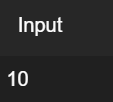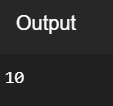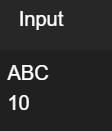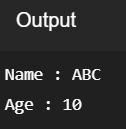# Input in C++

• Difficulty Level : Basic
• Last Updated : 01 Feb, 2021

The cin object in C++ is used to accept the input from the standard input device i.e., keyboard. it is the instance of the class istream. It is associated with the standard C input stream stdin. The extraction operator(>>) is used along with the object cin for reading inputs. The extraction operator extracts the data from the object cin which is entered using the keyboard.

Program 1:

Below is the C++ program to implement cin object to take input from the user:

## C++

 `// C++ program to demonstrate the ` `// cin object ` `#include ` `using` `namespace` `std; ` ` `  `// Driver Code ` `int` `main() ` `{ ` `    ``int` `i; ` ` `  `    ``// Take input using cin ` `    ``cin >> i; ` ` `  `    ``// Print output ` `    ``cout << i; ` ` `  `    ``return` `0; ` `}`

Input:Output:Note: Multiple inputs can also be taken using the extraction operators(>>) with cin.

Program 2:

Below is the C++ program to implement multiple inputs from the user:

## C++

 `// C++ program to demonstrate the taking ` `// multiple inputs from the user ` `#include ` `using` `namespace` `std; ` ` `  `// Driver Code ` `int` `main() ` `{ ` `    ``string name; ` `    ``int` `age; ` ` `  `    ``// Take multiple input using cin ` `    ``cin >> name >> age; ` ` `  `    ``// Print output ` `    ``cout << ``"Name : "` `<< name << endl; ` `    ``cout << ``"Age : "` `<< age << endl; ` ` `  `    ``return` `0; ` `}`

Input:Output:My Personal Notes arrow_drop_up
Recommended Articles
Page :